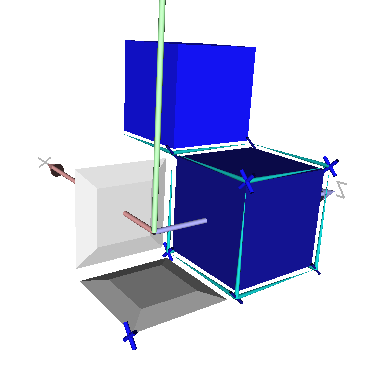DGtal  1.3.beta
topology/ctopo-1s-3d.cpp

A simple example of cellular grid space with several cells instantiated and visualized in 3D. This program outputs this image.

Cells may be unsigned or signed#include <iostream>
#include "DGtal/base/Common.h"
#include "DGtal/helpers/StdDefs.h"
#include "DGtal/io/viewers/Viewer3D.h"
#include "DGtal/io/DrawWithDisplay3DModifier.h"
using namespace std;
using namespace DGtal;
using namespace DGtal::Z3i;
int main( int argc, char** argv )
{
// for 3D display with Viewer3D
QApplication application(argc,argv);
Point plow(0,0,0);
Point pup(3,3,2);
Domain domain( plow, pup );
K.init( plow, pup, true );
MyViewer viewer(K);
viewer.show();
//viewer << SetMode3D( domain.className(), "Paving" );
SCell ptlow = K.sPointel( plow ); // pointel (0*2,0*2, 0*2)
SCell ptup1 = K.sPointel( pup ); // pointel (3*2,3*2, 2*2)
SCell ptup2 = K.sTranslation( ptup1, Point::diagonal() ); // pointel (4*2, 4*2, 3*2)
viewer << ptlow << ptup1 << ptup2;
// drawing cells of dimension 0
SCell p1= K.sCell(Point(0,0,2),false); // pointel (0*2,0*2,2*2)
SCell p2= K.sCell(Point(0,2,2)); // ...
SCell p3= K.sCell(Point(2,2,2),false);
SCell p4= K.sCell(Point(2,0,2));
SCell p5= K.sCell(Point(0,0,4),false);
SCell p6= K.sCell(Point(0,2,4));
SCell p7= K.sCell(Point(2,2,4), false);
SCell p8= K.sCell(Point(2,0,4));
viewer << p1 << p2 << p3 << p4 << p5 << p6 << p7 << p8;
// drawing Cells of dimension 1
SCell linel0 = K.sCell( Point( 1, 0, 2 ) ); // linel (2*1+1, 0, 2*2)
SCell linel1 = K.sCell( Point( 1, 2, 2 ) ); // ...
SCell linel2 = K.sCell( Point( 0, 1, 2 ) );
SCell linel3 = K.sCell( Point( 2, 1, 2 ) );
SCell linel4 = K.sCell( Point( 1, 0, 4 ) );
SCell linel5 = K.sCell( Point( 1, 2, 4 ) );
SCell linel6 = K.sCell( Point( 0, 1, 4 ) );
SCell linel7 = K.sCell( Point( 2, 1, 4 ) );
SCell linel8 = K.sCell( Point( 0, 0, 3 ) );
SCell linel9 = K.sCell( Point( 0, 2, 3 ) );
SCell linel10 = K.sCell( Point( 2, 0, 3 ) );
SCell linel11 = K.sCell( Point( 2, 2, 3 ) );
SCell linel12 = K.sCell( Point( 3, 2, 2 ) );
viewer << linel0<< linel1<< linel2 << linel3 ;
viewer << linel4<< linel5<< linel6 << linel7 ;
viewer << linel8<< linel9<< linel10 << linel11 << linel12;
// drawing cells of dimension 2
SCell surfelA = K.sCell( Point( 2, 1, 3 ) ); // surfel (2*2,2*1+1,2*3+1)
SCell surfelB = K.sCell( Point( 1, 0, 1 ) ); // surfel (2*1,2*0,2*1+1)
SCell surfelC = K.sCell( Point( 2, 1, 1 ),false ); // surfel (2*2,2*1+1,2*1+1)
viewer << surfelA << surfelB << surfelC;
// drawing cells of dimension 3
SCell vox1 = K.sCell( Point( 3, 3, 3 ) ); // voxel (2*3+1,2*3+1,2*3+1)
SCell vox2 = K.sCell( Point( 1, 1, 3 ) ,false ); // voxel (2*1+1,2*1+1,2*3+1)
viewer << vox1 << vox2;
viewer << CameraPosition(-2.9, 2.96, 2.64)
<< CameraUpVector(0.32, 0.900, 0.29);
return application.exec();
return 0;
}
DGtal::HyperRectDomain< Space >
DGtal::KhalimskySpaceND::sTranslation
SCell sTranslation(const SCell &p, const Vector &vec) const
Add the vector [vec] to [p].
DGtal::KhalimskySpaceND::init
bool init(const Point &lower, const Point &upper, bool isClosed)
Specifies the upper and lower bounds for the maximal cells in this space.
DGtal::KhalimskySpaceND::sCell
SCell sCell(const SPreCell &c) const
From a signed cell, returns a signed cell lying into this Khalismky space.
K
KSpace K
Definition: testCubicalComplex.cpp:62
DGtal::Display3D::updateDisplay
@ updateDisplay
Definition: Display3D.h:249
DGtal::SignedKhalimskyCell
Represents a signed cell in a cellular grid space by its Khalimsky coordinates and a boolean value.
Definition: KhalimskySpaceND.h:208
DGtal::Viewer3D< Space, KSpace >
DGtal
DGtal is the top-level namespace which contains all DGtal functions and types.
DGtal::KhalimskySpaceND::sPointel
SCell sPointel(Point p, Sign sign=POS) const
From the digital coordinates of a point in Zn, builds the corresponding pointel (cell of dimension 0)...
DGtal::Z3i
Z3i this namespace gathers the standard of types for 3D imagery.
CameraDirection class to set camera direction.
Definition: DrawWithBoard3DTo2DModifier.h:90
main
int main(int argc, char **argv)
Definition: testArithmeticDSS-benchmark.cpp:147
DGtal::CameraPosition
CameraPosition class to set camera position.
Definition: DrawWithBoard3DTo2DModifier.h:70
domain
Domain domain
Definition: testProjection.cpp:88
DGtal::PointVector< dim, Integer >
DGtal::Viewer3D::show
virtual void show()
Overload QWidget method in order to add a call to updateList() method (to ensure that the lists are w...
DGtal::CameraUpVector
CameraUpVector class to set camera up-vector.
Definition: DrawWithBoard3DTo2DModifier.h:110
Point
MyPointD Point
Definition: testClone2.cpp:383
DGtal::KhalimskySpaceND
Aim: This class is a model of CCellularGridSpaceND. It represents the cubical grid as a cell complex,...
Definition: KhalimskySpaceND.h:64